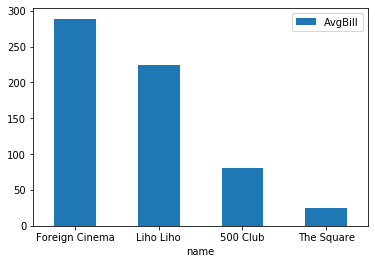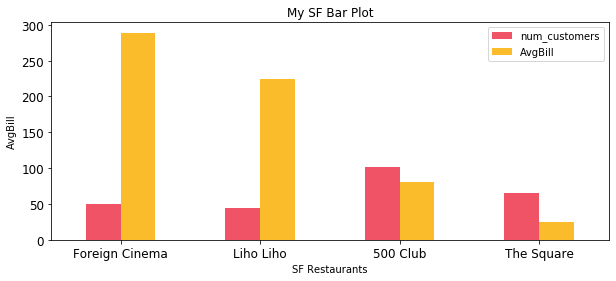Pandas Bar Plot is a great way to visually compare 2 or more items together. Traditionally, bar plots use the y-axis to show how values compare to each other.

In order to make a bar plot from your DataFrame, you need to pass a X-value and a Y-value. Pandas will draw a chart for you automatically.

`pandas.DataFrame.plot.bar(x=your_x_values, y=your_y_values)`

Pseudo Code: Construct a bar plot from a column(s) and index within a DataFrame.

## Pandas Bar Plot

### Resample Main Parameters

• x – What you want to have your bars be. This is traditionally a label (cat, dog, mouse).
• y – What you want the values or height of your bar plot to be. This is almost always a number (3, 4, 5, 6, etc.)
• color – The color you want your bars to be. If you have multiple sets of bars (like in a grouped or stacked bar plot) you can pass multiple colors via a list or dict.
• **kwargs – Pandas plot has a ton of general parameters you can pass. These are all agnostic to the type of plot you do.

Now the fun part, let’s take a look at a code sample

In :
```import pandas as pd
```

### Pandas Bar Plot¶

You can create a bar plot directly from your dataframe. Let's check out some examples:

1. Simple Bar Plot
2. Comparing two groups together
3. Stacked Bar Plot
4. Using Matplotlib format parameters

First, let's create our DataFrame

In :
```df = pd.DataFrame([('Foreign Cinema', 50, 289.0),
('Liho Liho', 45, 224.0),
('500 Club', 102, 80.5),
('The Square', 65, 25.30)],
columns=('name', 'num_customers', 'AvgBill')
)
df
```
Out:
0Foreign Cinema50289.0
1Liho Liho45224.0
2500 Club10280.5
3The Square6525.3

### 1. Simple Bar Plot¶

In order to create a bar plot, you need to pass a X and Y values. X will be your categories, or how you want to separate your bars. Y will be the value of your bars, or how high they are.

Note: Rot = Rotation. When I specify rot=0, I'm telling pandas not to rotate my x labels. What happens if you remove this?

In :
```df.plot.bar(x='name', y='AvgBill', rot=0);
```### 2. Comparing two groups together¶

Say you have two items you want to compare next to each other? This is called a grouped bar chart.

You can do this by passing all columns to your bar chart. Notice how I'm not specifying a Y, so pandas takes all other columns besides my X

In :
```df.plot.bar(x='name', rot=0);
```### 3. Stacked Bar Plot¶

Instead of having my bar plots groups side by side, I can also stack them on top of each other. You do this by setting stacked=True

In :
```df.plot.bar(x='name', stacked=True, rot=0);
```### 4. Using Matplotlib format parameters¶

As with any other Pandas Plot, you can take advantage of a full list of general plot parameters. Let's explore some.

In :
```df.plot.bar(x='name',
rot=0,
color=[(240/255,83/255,101/255), (250/255,188/255,42/255)], # passing normalized RGB colors
figsize=(10,4), # Figsize to make the plot larger
title='My SF Bar Plot', # Adding a title to the top
xlabel="SF Restaurants", # Adding a label on the xaxis
ylabel="AvgBill", # Adding a label on y axis
fontsize='large'); # Making my ticks a bit bigger
```